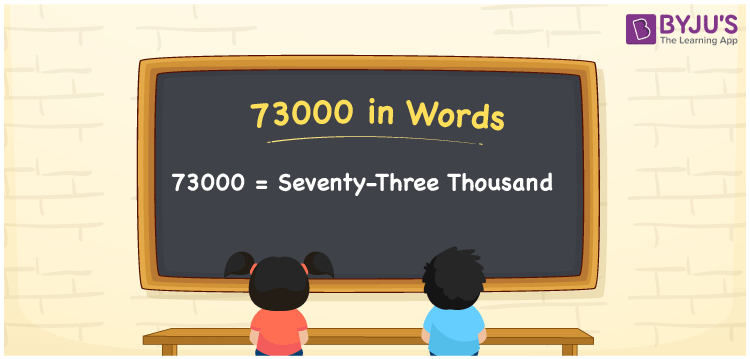# 73000 in Words

73000 in words is written as Seventy-three thousand. In both the International System of Numerals and the Indian System of Numerals, 73000 in words is written as Seventy-three thousand. The number 73000 is a Cardinal Number as it represents some quantity. For example, “he has 73000 rupees in his bank account”.

 73000 in Words Seventy-three thousand Seventy-three thousand in number 73000

## 73000 in English Words

73000 in English words is read as “Seventy-three thousand.”## How to Write 73000 in Words?

To write 73000 in words, we shall use the place value chart. In the place value chart, put 7 in the ten thousands, 3 in the thousands, and 0 in the hundreds, the tens and the ones, respectively. Let us make a place value chart to write the number 73000 in words.

 Ten Thousands Thousands Hundreds Tens Ones 7 3 0 0 0

Thus, we can write the expanded form as

7 × Ten Thousand + 3 × Thousand + 0 × Hundred + 0 × Ten + 0 × One

= 7 × 10000 + 3 × 1000 + 0 × 100 + 0 × 10 + 0 × 1

= 70000 + 3000 + 0 + 0 + 0

= 73000

= Seventy-three thousand.

73000 is a natural number between 72999 and 73001.

73000 in words – Seventy-three thousand

• Is 73000 an odd number? – No
• Is 73000 an even number? – Yes
• Is 73000 a perfect square number? – No
• Is 73000 a perfect cube number? – No
• Is 73000 a prime number? – No
• Is 73000 a composite number? – Yes

## Frequently Asked Questions on 73000 in Words

Q1

### How to write 73000 in words?

73000 in words is written as Seventy-three thousand.
Q2

### How to write 73000 in the International and Indian System of Numerals?

In both, the system of numerals, 73000 in words, is written as Seventy-three thousand.
Q3

### How to write 73000 in a place value chart?

In the place value chart, write 7 in the ten thousands, 3 in the thousands, and 0 in the hundreds, the tens and the ones, respectively.